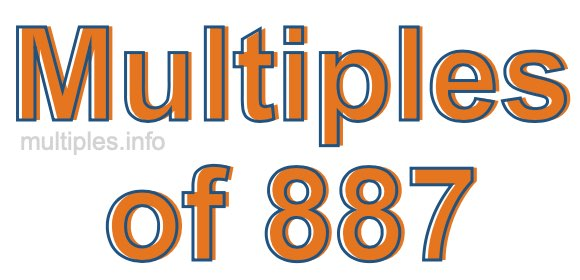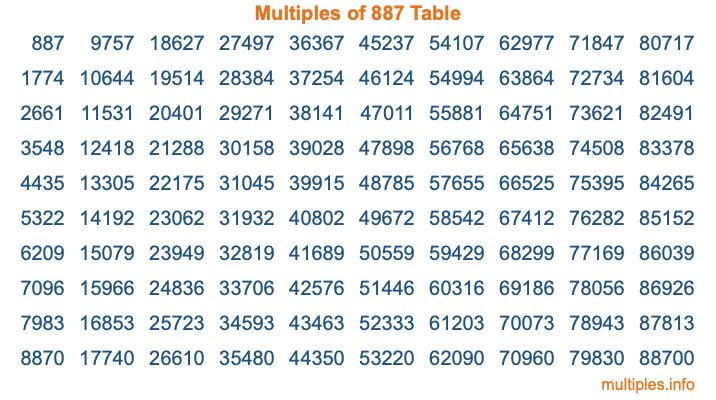Multiples of 887Welcome to the Multiples of 887 page. Here we will first teach you everything you will ever need to know about the multiples of 887, and then give you a study guide summary of everything we taught you to make sure you remember it all. Use this page to look up facts and learn information about the multiples of 887. This page will make you a multiples of eight hundred eighty-seven expert!

Definition of Multiples of 887
Multiples of 887 are all the numbers that when divided by 887 equal an integer. Each of the multiples of 887 are called a multiple. A multiple of 887 is created by multiplying 887 by an integer.

Therefore, to create a list of multiples of 887, you start with 1 multiplied by 887, then 2 multiplied by 887, then 3 multiplied by 887, and so on for as long as you want. Thus, the list of the first five multiples of 887 is 887, 1774, 2661, 3548, and 4435. To see a larger list of multiples of 887, see the printable image of Multiples of 887 further down on this page. We also have a category where you can choose any nth multiple of 887.

Multiples of 887 Checker
The Multiples of 887 Checker below checks to see if any number of your choice is a multiple of 887. In other words, it checks to see if there is any number (integer) that when multiplied by 887 will equal your number. To do that, we divide your number by 887. If the the quotient is an integer, then your number is a multiple of 887.

Is  a multiple of 887?

Least Common Multiple of 887 and ...
A Least Common Multiple (LCM) is the lowest multiple that two or more numbers have in common. This is also called the smallest common multiple or lowest common multiple and is useful to know when you are adding our subtracting fractions. Enter one or more numbers below (887 is already entered) to find the LCM.

Check out our LCM Calculator if you need more details about the Least Common Multiple or if you need the LCM for different numbers for adding and subtraction fractions.

nth Multiple of 887
As we stated above, 887 is the first multiple of 887, 1774 is the second multiple of 887, 2661 is the third multiple of 887, and so on. Enter a number below to find the nth multiple of 887.

th multiple of 887

Multiples of 887 vs Factors of 887
887 is a multiple of 887 and a factor of 887, but that is where the similarities end. All postive multiples of 887 are 887 or greater than 887. All positive factors of 887 are 887 or less than 887.

Below is the beginning list of multiples of 887 and the factors of 887 so you can compare:

Multiples of 887: 887, 1774, 2661, 3548, 4435, etc.

Factors of 887: 1, 887

As you can see, the multiples of 887 are all the numbers that you can divide by 887 to get a whole number. The factors of 887, on the other hand, are all the whole numbers that you can multiply by another whole number to get 887.

It's also interesting to note that if a number (x) is a factor of 887, then 887 will also be a multiple of that number (x).

Multiples of 887 vs Divisors of 887
The divisors of 887 are all the integers that 887 can be divided by evenly. Below is a list of the divisors of 887.

Divisors of 887: 1, 887

The interesting thing to note here is that if you take any multiple of 887 and divide it by a divisor of 887, you will see that the quotient is an integer.

Multiples of 887 Table
Below is an image of the first 100 multiples of 887 in a table. The table is in chronological order, column by column. The first column has the first ten multiples of 887, the second column has the next ten multiples of 887, and so on.The Multiples of 887 Table is also referred to as the 887 Times Table or Times Table of 887. You are welcome to print out our table for your studies.

Negative Multiples of 887
Although not often discussed or needed in math, it is worth mentioning that you can make a list of negative multiples of 887 by multiplying 887 by -1, then by -2, then by -3, and so on, to get the following list of negative multiples of 887:

-887, -1774, -2661, -3548, -4435, etc.

Multiples of 887 Summary
Below is a summary of important Multiples of 887 facts that we have discussed on this page. To retain the knowledge on this page, we recommend that you read through the summary and explain to yourself or a study partner why they hold true.

There are an infinite number of multiples of 887.

A multiple of 887 divided by 887 will equal a whole number.

887 divided by a factor of 887 equals a divisor of 887.

The nth multiple of 887 is n times 887.

The largest factor of 887 is equal to the first positive multiple of 887.

887 is a multiple of every factor of 887.

887 is a multiple of 887.

A multiple of 887 divided by a divisor of 887 equals an integer.

887 divided by a divisor of 887 equals a factor of 887.

Any integer times 887 will equal a multiple of 887.

Multiples of a Number
Here you can get the multiples of another number, all with the same attention to detail as we did for multiples of 887 on this page.

Multiples of
Multiples of 888
Did you find our page about multiples of eight hundred eighty-seven educational? Do you want more knowledge? Check out the multiples of the next number on our list!